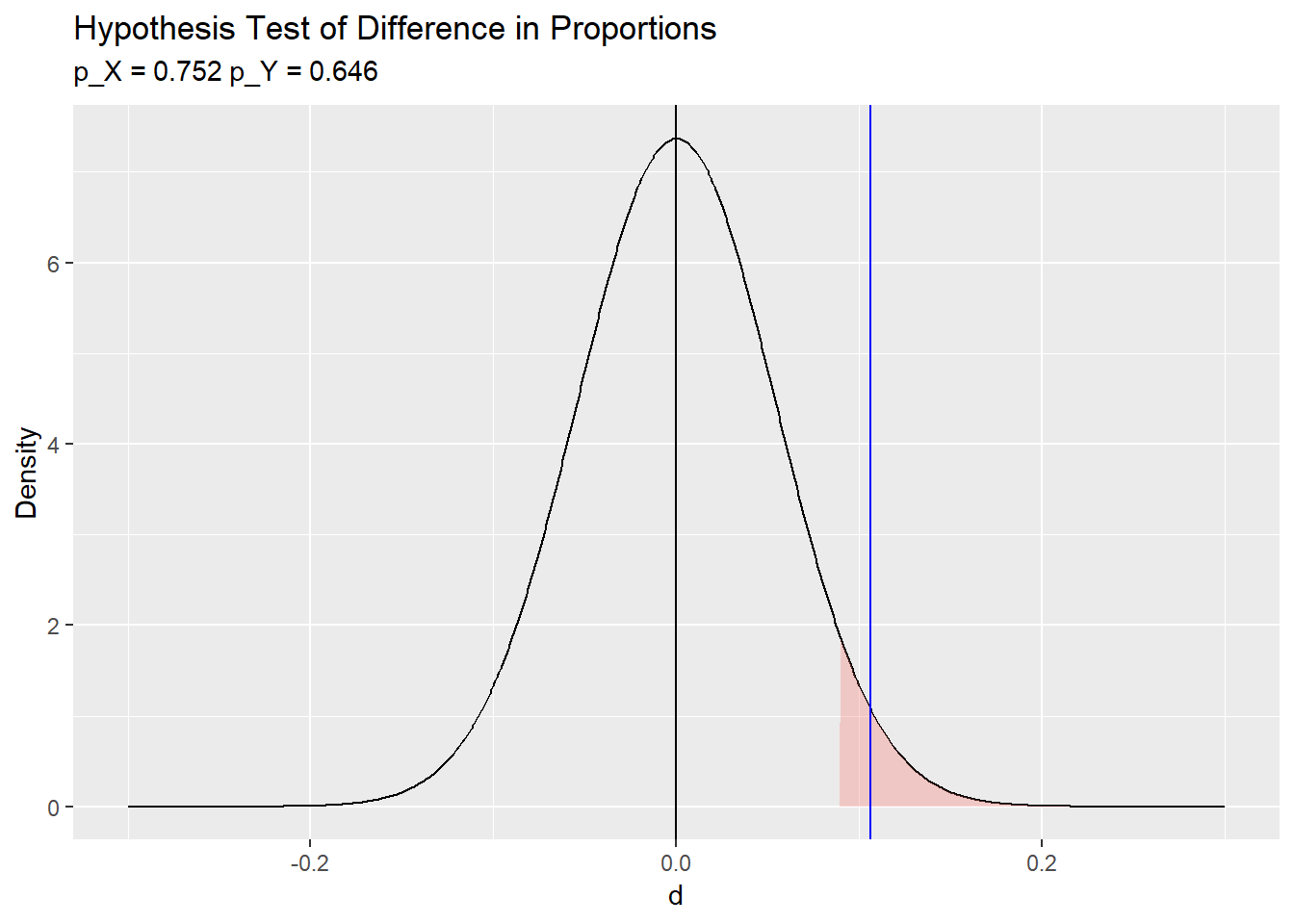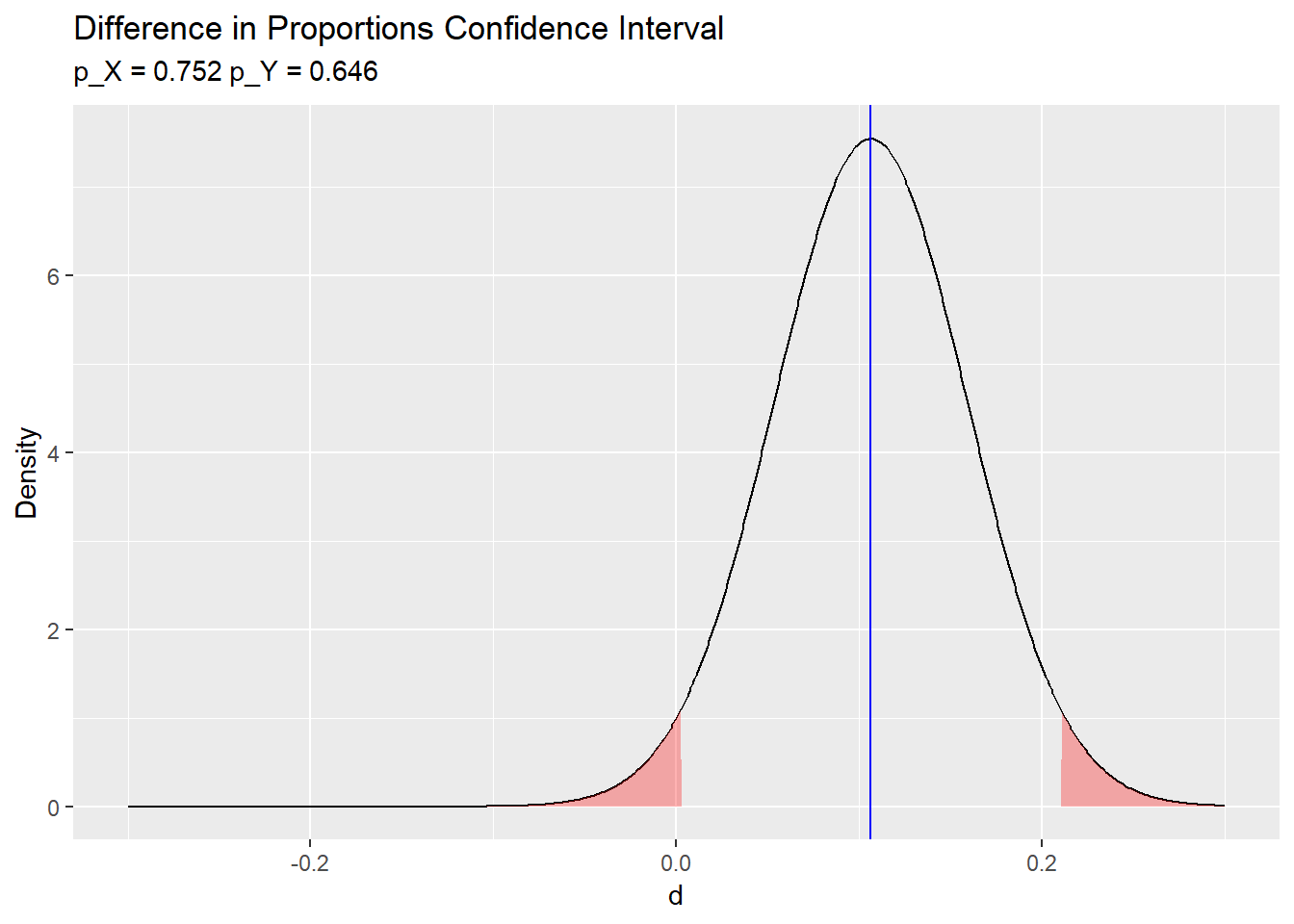# Difference in Proportions CI and Test

### Calculating confidence intervals and conducting hypothesis tests for the difference in proportions of two independent categorical variables using R.

#### 2019-02-25

The proportion difference test uses the difference in sample proportions $$\hat{d} = p_X - p_Y$$Y as an estimate of the difference in population proportions $$d = \pi_X - \pi_Y$$ to evaluate an hypothesized difference in population proportions $$d_0 = \pi_{X_0} - \pi_{Y_0}$$. The null hypothesis is $$H_0: d = d_0$$ and the alternative hypothesis is $$H_a: d \ne d_0$$, $$H_a: d < d_0$$, or $$H_a: d > d_0$$. One can also construct a $$(1 - \alpha)\%$$ confidence interval around $$\hat{d}$$ to estimate $$d$$ within a margin of error $$\epsilon$$.1 See PSU STAT 500 online material, and Stat Trek.

The normal approximation method applies when the central limit theorem conditions hold:

• the sample is independently drawn (random sampling without replacement from $$n < 10\%$$ of the population in observational studies, or random assignment in experiments),
• there are at least $$n_i p_i >= 5$$ successes and $$n_i (1 - p_i) >= 5$$ failures for each group $$i \in (X,Y)$$,
• the sample sizes are both $$>=30$$, and
• the probability of success for each group is not extreme, $$(0.2, 0.8)$$.

Under the normal approximation method, the sampling distribution of the difference in population proportions has a normal distribution centered at the measured difference in proportions $$\hat{d} = p_X - p_Y$$Y with standard error $$se = \sqrt{\frac{p_X (1 - p_X)}{n_X} + \frac{p_Y (1 - p_Y)}{n_Y}}$$. The measured difference in proportions $$\hat{d}$$ and standard deviation $$s$$ approximate the population difference in proportions $$d = \pi_X - \pi_Y$$ and standard deviation $$\sigma$$. Test $$H_0: d = d_0$$ with test statistic $$Z = \frac{\hat{d} - d_0}{se_{d_0}}$$ where $$se_{d_0} = \sqrt{p (1 - p) \frac{1}{n_X} + \frac{1}{n_Y}}$$ is the standard error of the hypothesized population proportion sampling distribution and $$p$$ is the pooled proportion $$p = \frac{x+y}{n_X + n_Y}$$.[Why do we pool the proporitions for the hypothesis test, but not for the CI?] Define a $$(1 - \alpha)\%$$ confidence interval as $$\hat{d} \pm z_{\alpha {/} 2} se$$ where $$z_{\alpha {/} 2} se = \epsilon$$ is the margin of error.

## Example

In an experiment on the effectiveness of computer training, $$n_X = 125$$ students were assigned to computer training and $$n_Y = 175$$ were assigned to traditional training. $$x = 94 (75.2 \%)$$ of the computer training students passed the certification exam and $$y = 113 (64.6 \%)$$ of the tradition training students passed the certification exam, a difference of $= .752 - .646 = .1063. At an $$\alpha = 0.05$$ level of significance, does computer training produce higher success rates than traditional training? What is the $$(1 - \alpha) = 95%$$ confidence interval of the difference in success rates? x <- 94 n_x <- 125 y <- 113 n_y <- 175 (p_x <- x / n_x) ##  0.752 (p_y <- y / n_y) ##  0.6457143 (d <- (p_x - p_y)) ##  0.1062857 (d_hat <- p_x - p_y) ##  0.1062857 alpha <- 0.05 The samples are independently drawn because the experiment is random assignment. There are $$>= 5$$ success, $$>= 5$$ failures, $$>= 30$$ observations, and $$.2 < p <.8$$ probability of success for both $$X$$ and $$Y$$, so we can use the difference in proportions test. The null hypothesis is $$H_0: d = d_0 = (\pi_x - \pi_y) = 0$$ with alternative hypothesis $$H_a: d > 0$$. This is a right-tail test. The test statistic is $$Z = \frac{d - d_0}{se_{d_0}}$$ where $$se_{d_0} = \sqrt{p (1 - p) \frac{{1}}{{n_X}} + \frac{{1}}{{n_Y}}}$$ with $$p = \frac{{x+y}}{{n_X + n_Y}} = \frac{94 + 113}{125 + 175} = 0.69$$. $$se_{d_0} = \sqrt{.69 (1 - .69) \frac{{1}}{{125}} + \frac{{1}}{{175}}} = .0542$$. $$Z = \frac{.1063 - 0}{.0542} = 1.96$$. $$P(z > 1.96) = .0249$$, so reject $$H_0$$ at the $$\alpha = 0.05$$ level of significance. d_0 <- 0 (p <- (x + y) / (n_x + n_y)) ##  0.69 (se_d0 <- sqrt(p * (1 - p) * (1 / n_x + 1 / n_y))) ##  0.05416166 (z = (d_hat - d_0) / se_d0) ##  1.962379 pnorm(q = d, mean = d_0, sd = se_d0, lower.tail = FALSE) ##  0.02485918 R package stats function prop.test does this same calculation. (prop.test.result <- prop.test(x = c(x, y), n = c(n_x, n_y), alternative = "greater", conf.level = 1 - alpha, correct = FALSE)) ## ## 2-sample test for equality of proportions without continuity ## correction ## ## data: c(x, y) out of c(n_x, n_y) ## X-squared = 3.8509, df = 1, p-value = 0.02486 ## alternative hypothesis: greater ## 95 percent confidence interval: ## 0.01926052 1.00000000 ## sample estimates: ## prop 1 prop 2 ## 0.7520000 0.6457143 Graph the hypothesis test with rejection range in red. library(ggplot2) library(dplyr) # for %>% lrr = -Inf urr = qnorm(p = alpha, mean = d_0, sd = se_d0, lower.tail = FALSE) data.frame(d = -300:300 / 1000) %>% mutate(density = dnorm(x = d, mean = d_0, sd = se_d0)) %>% mutate(rr = ifelse(d < lrr | d > urr, density, 0)) %>% ggplot() + geom_line(aes(x = d, y = density)) + geom_area(aes(x = d, y = rr, fill = "red"), alpha = 0.3) + geom_vline(aes(xintercept = d_hat), color = "blue") + geom_vline(aes(xintercept = d_0), color = "black") + labs(title = bquote("Hypothesis Test of Difference in Proportions"), subtitle = bquote('p_X ='~.(round(p_x,3))~'p_Y ='~.(round(p_y,3))), x = "d", y = "Density") + theme(legend.position="none")Define a $$(1 - \alpha)\%$$ confidence interval as $$\hat{d} \pm z_{\alpha {/} 2} se$$ where $$z_{\alpha {/} 2} se = \epsilon$$ is the margin of error. $$se = \sqrt{\frac{p_X (1 - p_X)}{n_X} + \frac{p_Y (1 - p_Y)}{n_Y}} = \sqrt{\frac{.752 (1 - .752)}{125} + \frac{.646 (1 - .646)}{175}} = .0529$$ and $$z_{\alpha {/} 2} = 1.96$$. (se <- sqrt(p_x * (1 - p_x) / n_x + p_y * (1 - p_y) / n_y)) ##  0.05290756 (z_crit <- qnorm(p = alpha / 2, mean = 0, sd = 1, lower.tail = FALSE)) ##  1.959964 lcl <- d_hat - z_crit * se ucl <- d_hat + z_crit * se cat("95% CI: ", round(d_hat, 4), "+/-", round(se * z_crit, 4), "= (", round(lcl, 4), ", ", round(ucl, 4), ")") ## 95% CI: 0.1063 +/- 0.1037 = ( 0.0026 , 0.21 ) R function prop.test can calculate a confidence interval around a proportion. In this case, use a two-tail distribution. (prop.test.result <- prop.test(x = c(x, y), n = c(n_x, n_y), conf.level = 1 - alpha, correct = FALSE)) ## ## 2-sample test for equality of proportions without continuity ## correction ## ## data: c(x, y) out of c(n_x, n_y) ## X-squared = 3.8509, df = 1, p-value = 0.04972 ## alternative hypothesis: two.sided ## 95 percent confidence interval: ## 0.002588801 0.209982628 ## sample estimates: ## prop 1 prop 2 ## 0.7520000 0.6457143 Graph the 95% CI. lcl <- round(prop.test.result$conf.int, 3)
ucl <- round(prop.test.result\$conf.int, 3)
data.frame(d = -300:300 / 1000) %>%
mutate(density = dnorm(x = d, mean = d_hat, sd = se)) %>%
mutate(rr = ifelse(d < lcl | d > ucl, density, 0)) %>%
ggplot() +
geom_line(aes(x = d, y = density)) +
geom_area(aes(x = d, y = rr), fill = "red", alpha = 0.3) +
geom_vline(aes(xintercept = d_hat), color = "blue") +
labs(title = bquote("Difference in Proportions Confidence Interval"),
subtitle = bquote('p_X ='~.(round(p_x,3))~'p_Y ='~.(round(p_y,3))),
x = "d",
y = "Density") +
theme(legend.position="none")